Courses

# Previous year questions (2016-20) - Units and Measurements Class 11 Notes | EduRev

## JEE : Previous year questions (2016-20) - Units and Measurements Class 11 Notes | EduRev

The document Previous year questions (2016-20) - Units and Measurements Class 11 Notes | EduRev is a part of the JEE Course Physics For JEE.
All you need of JEE at this link: JEE

Q.1. The dimensions of B2/2μ0, where B is magnetic field and μ0 is the magnetic permeability of vacuum, is     [(JEE Main 2020)]
(a) [MLT−2]
(b) [ML2T−1]
(c) [ML2T−2]
(d) [ML−1T−2]
Ans.
(d)
Solution: The given quantity is energy density of magnetic field.
ε = E/V = B2/2μ0
So, dimension is given by
[B2/2μ0] = [ML2T2/L3] = [ML-1T-2]

Q.2. The dimensions of stopping potential V0 in photoelectric effect in the units of Planck’s constant ‘h’, speed of light ‘c’ and Gravitational constant ‘G’ and ampere 'A' is    [(JEE Main 2020)]
(a) h1/3G2/3 c1/3A-1
(b) h2/3G5/3 c1/3 A-1
(c) h-2/3c-1/3G4/3A-1
(d) h2 G3/2 c1/3 A-1
Ans.
None
Solution: We have
v0 = hwcxGyAz
We know that
V0 = [ML2T-3A-1], h = [ML2T-1]
c = [LT-1], G = [M-1L3T-2], I = [A]
So,
[ML2T-3A-1] = [ML2T-1]w[LT-1]x[M-1L3T-2]y[A]z
⇒ [ML2T-3A-1] = [Mw-yL2w+x+3yT-w-x-2yAz]
⇒ w - y = 1, 2w + x + 3y = 2, -w-x-2y = -3, z = -1
Solving the equations, we get
w = 0, x = 5, y = -1, z = -1
Therefore,
V= h0c5G-1A-1

Q.3. A simple pendulum is being used to determine the value of gravitational acceleration g at a certain place. The length of the pendulum is 25.0 cm and a stopwatch with 1s resolution measures the time taken for 40 oscillations to be 50 s. The accuracy in measurement of g is    [(JEE Main 2020)]
(a) 5.40%
(b) 3.40%
(c) 4.40%
(d) 2.40%
Ans.
(c)
Solution: Given that
L = 25cm, Δt = 1s, n = 40, t= 50s
Time period of simple pendulum is given by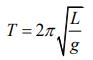⇒ g = 4π2L/t2
⇒ Δg/g = (ΔL/L + 2Δt/t) = (0.1/25.0) + (2 1/50) = (1.1/25)
⇒ (Δg/g) x 100% = 4.4%

Q.4. A quantity f is given by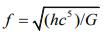where 'c' is speed of light, 'G' universal gravitational constant and 'h' is the Planck’s constant. Dimension of f is that of    [(JEE Main 2020)]
(a) Area
(b) Energy
(c) Momentum
(d) Volume
Ans.
(b)
Solution: Given thatWe know that
h = [ML2T-1]
c = [LT-1]
G = [M-1L3T-2]
So,
f = [ML2T-1]1/2[LT-1]5/2[M-1L3T-2]-1/2
= [M1/2+1/2L2/2+5/2-3/2T-1/2-5/2+2/2] = [M1L2T-2]
The dimension is equal to energy dimension.

Q.5. If the screw on a screw-gauge is given six rotations, it moves by 3 mm on the main scale. If there are 50 divisions on the circular scale, the least count of the screw gauge is     [(JEE Main 2020)]
(a) 0.001 mm
(b) 0.02 mm
(c) 0.01 cm
(d) 0.001 cm
Ans.
(a)
Solution: Given that
6 rotations = 3 mm MSR (Main Scale Reading)
⇒ 1 rotation = 1/2 mm = 0.5 mm
Number of divisions in circular scale = 50 divisions
Least count of screw-gauge is given by
LC = (MSR in 1 rotation/circular division) = 0.5/50 mm = 0.001mm

Q 6. Four sets of three measured physical quantities are given below. Which of the following options is correct?     [(JEE Main 2020)]
(i) A1 = 24.36, B1 = 0.0724, C1 = 256.2
(ii) A2 = 24.44, B2 = 16.082, C1 = 240.2
(iii) A3 = 25.2, B3 = 19.2812, C3 = 236.183
(iv) A4 = 25, B4 = 236.191, C4 = 19.5

(1) A4 + B4 + C4 < A1 + B1 + C1 < A3 + B3 + C3 < A2 + B2 + C2
(2) A1 + B1 + C1 = A2 + B2 + C2 = A3 + B3 + C3 = A4 + B4 + C4
(3) A4 + B4 + C4 < A1 + B1 + C1 = A2 + B2 + C2 = A3 + B3 + C3
(4) A1 + B1 + C1 < A3 + B3 + C3 < A2 + B2 + C2 < A4 + B4 + C4
Ans.
None
Solution: Given that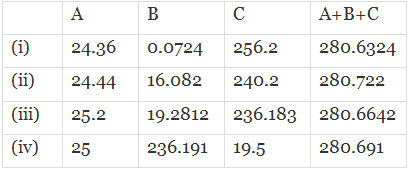So, we have A1+B1 +C1<A3+B3+C3 < A4+B4+C4<A2+B2+C2

Q.7. Expression for time in terms of G (universal gravitational constant), h (Planck constant) and c (speed of light) is proportional to    [(JEE Main 2019)]
(a)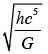(b)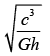(c)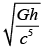(d)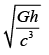Ans.
(c)
Solution: Dimension of time, t = [T]
Now,
t ∝ Gkhqcr
t = kGkhqcr  ....(1)
Dimension of Gravitational constant, G = [M−1L3T−2]
Dimension of Planck’s constant h = [ML2T−1]
Dimension of speed of light c = [LT−1]
Put the Dimensions in Eq.(1), we get
[T] = [M-1L3T-2]p[ML2T-1]q[LT-1]r
[T] = [M-p+q][L3p+2q+r][T-2p-q-r]
On comparing the power of both sides:
-p + q = 0 ⇒ p = q   ....(2)
3p + 2q + r = 0 ⇒ 5p + r = 0    ....(3)
-2p - q - r = 1 ⇒ -3p - r = 1  ....(4)
On solving the above Eq. (2), (3) and (4), we get
p = q = 1/2 and r = -5/2
Put these values in Eq. (1), we get
t = G1/2h1/2c-5/2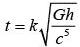where, k = constant

Q.8. The pitch and the number of divisions, on the circular scale, for a given screw gauge are 0.5 mm and 100, respectively. When the screw gauge is fully tightened without any object, the zero of its circular scale lies 3 divisions below the mean line. The readings of the main scale and the circular scale, for a thin sheet, are 5.5 mm and 48 respectively, the thickness of this sheet is    [JEE Main 2019]
(a) 5.755 mm
(b) 5.950 mm
(c) 5.725 mm
(d) 5.740 mm
Ans.
(c)
Solution: Least count = (Pitch/Number of division)
= 0.5/100
= 0.5 × 10-2 mm
Positive error = 3 × 0.5 × 10−2
= 1.5 × 10−2
= 0.015 mm Reading = MSR + CSR – Positive Error
= 5.5 × (48 × 0.5 × 10−2) – 0.015
= 5.5 + 0.24 – 0.015
= 5.725 mm

Q.9. The density of a material in SI units is 128 kg/m3. In certain units in which the unit of length is 25 cm and the unit of mass is 50 g, the numerical value of density of the material is     [JEE Main 2019]
(a) 40
(b) 16

(c) 640
(d) 410
Ans.
(a)
Solution: Density = Mass/Voulme
=[M]/[L3]
= [ML-3]
128 kg/m2 = h[50/1000]x[25/100]-3
= h × (1/20) × (4)3
⇒ h = (128 × 20)/64 = 40 unit

Q.10. The diameter and height of a cylinder are measured by a meter scale to be 12.6 ± 0.1 cm and 34.2 ± 0.1 cm, respectively. What will be the value of its volume in appropriate significant figures?   [JEE Main 2019]
(a) 4264 ± 81 cm3
(b) 4264.4 ± 81.0 cm3
(c) 4260 ± 80 cm3
(d) 4300 ± 80 cm3
Ans.
(c)
Solution: Diameter, D + ΔD = 12.6 ± 0.1 cm
height, h + Δh = 34.2 ± 0.1 cm
Volume of cylinder, V = πr2h
= πd2h/4
= (π/4) × (12.6)2 × 34.2
= 4262.229 = 4260
⇒ ΔV/V = 2dD/D + Δh/h
⇒ ΔV = [2 × (0.1/12.6) + (0.1/34.2] × 4266
⇒ ΔV = 80
⇒ V + ΔV = 4260 ± 80 cm3

Q.11. The force of interaction between two atoms is given by F = αβ exp(-x2/αkt), where x is the distance, k is the Boltzmann constant, T is temperature and α and β are two constants. The dimension of β is    (JEE Main 2019)
(a) MLT−2
(b) M2L2T−2
(c) M0L2T−4
(d) M2LT−4
Ans.
(d)
Solution: Given,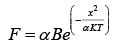⇒ k2/αKT = dimensionless
Dimension of α,
[α] = x2/KT = [L2]/[ML2T-2]
⇒ [α] = [M-1T2]
Dimension of F = Dimension of α × Dimension of β
Therefore, dimension of β = (Dimension of F/Dimension of α)
= [MLT-2]/[M-1T+2]
= [M2LT-4]

Q.12. If speed (V) , acceleration (A) and force (F) are considered as fundamental units, the dimension of Young’s modulus will be     [JEE Main 2019]
(a) V−2A2F−2
(b) V−2A2F2
(c) V−4A−2F
(d) V−4A2F
Ans.
(d)
Solution: Young's modulus Y = (F/A x L/L0)
Dimension of Young’s modulus = [ML−1T−2]
Now,
Y ∝ Va Ab Fc
⇒ Y = KVaAbFc
⇒ [ML-1T-2] = [LT-1]a[LT-2]b [MLT-2]c
⇒ [ML-1T-2] = [Mc][La+b+c][T-a-2b-2c]
Comparing both the sides, we get
⇒ c = 1
⇒ a + b + c = −1
⇒ −a – 2b – 2c = −2
By solving, we get
a = −4, b = 2, c = 1
Therefore, Y = kV−4 A2F1
⇒ Y = V−4A2F

Q.13. The least count of the main scale of a screw gauge is 1 mm. The minimum number of divisions on its circular scale required to measure 5 μm diameter of a wire is     [JEE Main 2019]
(a) 50
(b) 200
(c) 100
(d) 500
Ans.
(b)
Solution: We have,
Least count = 5 μm = 5 × 10−6 m
Pitch = 1 mm = 10−3 m
Thus, least count = (Pitch/Number of divisions on circular scale)
⇒ 5 × 10-6 = (10-3/No. of division on circular scale)
Therefore, number of divisions on circular scale = (10-3/5 × 10-6)
= 1000/5 = 200

Q.14. Let l, r, c and v represent inductance, resistance, capacitance and voltage, respectively. The dimension of 1/rcv in SI units will be    [JEE Main 2019]
(a) [LA−2]
(b) [A−1]
(c) [LTA]
(d) [LT2]
Ans.
(b)
Solution: Dimension of inductance = [M1L2T−2A−2] = [l]
Dimension of capacitance = [M−1L−2T4A2] = [c]
Dimension of resistance = [M1L2T−3A−2] = [r]
Dimension of voltage = [M1L2T−3A−1] = [v]
Dimension of l/rcv = [M1L2T-2A-2] [M-1L2T4A2][M1L2T-3A-2][M1L2T-3A-1]
= [ML2T-2A-2]/[ML2T-2A-1]
= [A-1]

Q.15. The density of a material in the shape of a cube is determined by measuring three sides of the cube and its mass. If the relative errors in measuring the mass and length are respectively 1.5% and 1%, the maximum error in determining the density is:    [JEE Main 2018]
(a) 2.5%
(b) 3.5%
(c) 4.5%
(d) 6%
Ans.
(c)
Solution: p = M/L3
Δp/p = (ΔM/Δ + 3ΔL/L)
= 1.5 + 3 × 1
= 4.5%

Q.16. In a screw gauge, 5 complete rotations of the screw cause it to move a linear distance of 0.25 cm. There are 100 circular scale divisions. The thickness of a wire measured by this screw gauge gives a reading of 4 main scale divisions and 30 circular scale divisions. Assuming negligible zero error, the thickness of the wire is:    [JEE Main 2018]
(a) 0.4300 cm
(b) 0.0430 cm
(c) 0.2150 cm
(d) 0.3150 cm
Ans.
(c)
Solution: Given:
5 complete rotations of screw = 0.25 cm
So 1 rotation of screw = 0.05
Hence, 1 main scale division = 0.05 cm
and 1 circular scale = 0.05/100 division = 5 × 10−4 cm.
Now Reading is 4 main scale and 30 circular scale divisions
So , thickness  = 4 × 0.05 + 30 × 5 × 10−4
= 0.2150 cm.

Q.17. The relative error in the determination of the surface area of a sphere is α. Then the relative error in the determination of its volume is:     [JEE Main 2018]
(a) 5/2 α
(b) 2/3 α
(c) α
(d) 3/2 α
Ans.
(d)
Solution: Area of sphere (A) = 4πR2
Taking log both sides:
ln A = In(4π) + 2In(R)
differentiating both sides
⇒ dA/A = 2dR/R
so, α = 2dR/R
⇒ dR/R = α/2
now, similarly
⇒ dv/v = 3dR/R
So, dv/v = 3α/2

Q.18. The percentage errors in quantities P, Q, R and S are 0.5%, 1%, 3% and 1.5% respectively in the measurement of a physical quantity A = P3Q2/√RS. The maximum percentage error in the value of A will be:    [JEE Main 2018]

(a) 6.5%
(b) 7.5%
(c) 6.0%
(d) 8.5%
Ans.
(a)
Solution: ΔA/A = 3ΔP/P + 2ΔQ/Q + 1/2(ΔR/R) + ΔS/S
= 3 × 0.5 + 2 × 1 + 1/2 × 3 + 1.5
= 1.5 + 2 + 1.5 + 1.5
ΔA/A = 6.5%

Q.19. The following observations were taken for determining surface tension T of water by capillary method:
Diameter of capillary, D = 1.25 × 10–2 m
Rise of water, h = 1.45 × 10–2 m.
Using g = 9.80 m/s2 and the simplified relation T= (rhg/2) x 103 N/m, the possible error in surface tension is closest to     [JEE Main 2017]
(a) 2.4%
(b) 10%
(c) 0.15%
(d) 1.5%
Ans.
(d)
Solution: (ΔT/T) × 100 = (ΔD/D) × 100 + (Δh/h) × 100
= (0.01/1.25) × 100 +(0.01/1.45) × 100
= (100/125) + (100/145)
= 0.8 + 0.689= 1.489
≈ 1.5%

Q.20. Time (T), velocity (3) and angular momentum (h) are chosen as fundamental quantities instead of mass, length and time. In terms of these, the dimensions of mass would be:    [JEE Main 2017]
(a) [M] = [T–1 C–2h]
(b) [M] = [T C–2h]
(c) [M] = [T–1C–2h–1]
(d) [M] = [T–1 C2h]
Ans.
(a)
Solution: M ∝ Tx vy hz
M1 L0T0 = (T)x(L1T-1)y(M1L2T-1)z
M1L0T0 = Mz Ly+2z + Tx-y-z
z = 1
y + 2z = 0   x - y - z = 0
y = -2
x + 2 - 1 = 0
x = -1
M ⇒ T-1 C-2 h1

Q.21. A physical quantity P is described by the relation P = a1/2 b2 c3 d–4 If the relative errors in the measurement of a,b,c and d respectively, are 2%, 1%, 3% and 5%, then the relative error in P will be-    [JEE Main 2017]
(a) 12%
(b) 8%
(c) 25%
(d) 32%
Ans.
(d)
Solution: ΔP/P = (1/2)(Δa/a) + 2(Δb/b) + 3(Δc/c) + 4(Δd/d)
= ((1/2) × 2) + (2 × 1) + (3 × 3) + (4 × 5)
= 32%

Q.22. A student measures the time period of 100 oscillations of a simple pendulum four times. The data set is 90 s, 91 s, 95 s and 92 s. If the minimum division in the measuring clock is 1 s, then the reported mean time should be:    [JEE Main 2016]
(a) 92 ± 2 s

(b) 92 ± 5.0 s
(c) 92 ± 1.8 s
(d) 92 ± 3 s
Ans.
(a)
Solution: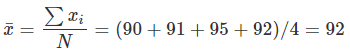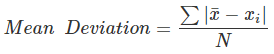= (2 + 1 + 3 + 0)/4 = 1.5
L. C. = 1 s.
∴ Required value = 92 ± 2 s

Q 23. A screw gauge with a pitch of 0.5 mm and a circular scale with 50 divisions is used to measure the thickness of a thin sheet of Aluminium. Before starting the measurement, it is found that when the two jaws of the screw gauge and brought in contact, the 45th division coincides with the main scale line and that the zero of the main scale is barely visible. What is the thickness of the sheet if the main scale reading is 0.5 mm and the 25th division coincides with the main scale line?    [JEE Main 2016]
(a) 0.75 mm
(b) 0.80 mm
(c) 0.70 mm
(d) 0.50 mm
Ans.
(b)
Solution: L.C. = (pitch/No. of division on circular scale) = 0.5/50 = 0.001 mm
-ve zero error = - 5 × L.C. = -0.005 mm
∴ Measured value
= 0.5 mm + {25 × 0.001 - (-0.05)} mm  = 0.80 mm

Q.24. In the following 'I' refers to current and other symbols have their usual meaning choose the option that corresponds to the dimensions of electrical conductivity:    [JEE Main 2016]
(a) M–1 L–3 T3 I2
(b) M–1 L3 T3 I
(c) ML–3 T–3 I2
(d) M–1 L –3 T3 I
Ans.
(a)
Solution: J = σE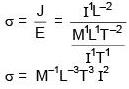Q.25. A,B,C and D are four different physical quantities having different dimensions. None of them is dimensionless. But we know that the equation AD = Cln (BD) holds true. Then which of the combination is not a meaningful quantity?    [JEE Main 2016]
(a)
A2 – B2 C2
(b)
(A/B) - C
(c)
(d)
(A-C)/D
Ans.
(d)
(B) (D) → dimensionless
Checking options one by one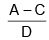Dimensions of A are not same as dimensions of C.

Offer running on EduRev: Apply code STAYHOME200 to get INR 200 off on our premium plan EduRev Infinity!

## Physics For JEE

187 videos|516 docs|263 tests

,

,

,

,

,

,

,

,

,

,

,

,

,

,

,

,

,

,

,

,

,

;